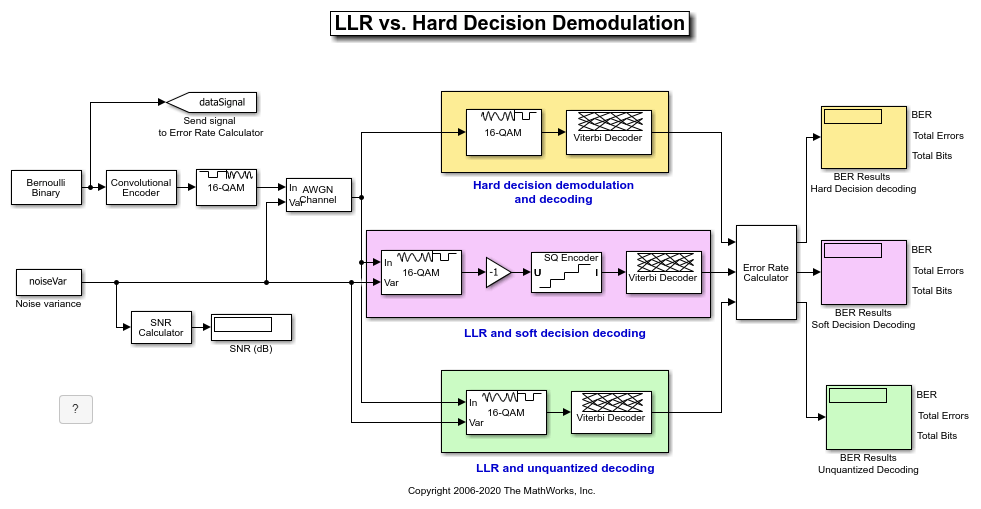# LLR vs. Hard Decision Demodulation in Simulink

This model shows the improvement in BER performance when using log-likelihood ratio (LLR) instead of hard decision demodulation in a convolutionally coded communication link.

For a MATLAB™ version of this example, see Log-Likelihood Ratio (LLR) Demodulation.

### System Setup

This example model simulates a convolutionally coded communication system having one transmitter, an AWGN channel and three receivers. The convolutional encoder has a code rate of 1/2. The system employs a 16-QAM modulation. The modulated signal passes through an additive white Gaussian noise channel. The top receiver performs hard decision demodulation in conjunction with a Viterbi decoder that is set up to perform hard decision decoding. The second receiver has the demodulator configured to compute log-likelihood ratios (LLRs) that are then quantized using a 3-bit quantizer. It is well known that the quantization levels are dependent on noise variance for optimum performance . The exact boundaries of the quantizer are empirically determined here. A Viterbi decoder that is set up for soft decision decoding processes these quantized values. The LLR values computed by the demodulator are multiplied by -1 to map them to the right quantizer index for use with Viterbi Decoder. To compute the LLR, the demodulator must be given the variance of noise as seen at its input. The third receiver includes a demodulator that computes LLRs which are processed by a Viterbi decoder that is set up in unquantized mode. The BER performance of each receiver is computed and displayed.

```modelName = 'commLLRvsHD'; open_system(modelName); ```### System Simulation and Visualization

Simulate this system over a range of information bit Eb/No values. Adjust these Eb/No values for coded bits and multi-bit symbols to get noise variance values required for the AWGN block and Rectangular QAM Baseband Demodulator block. Collect BER results for each Eb/No value and visualize the results.

```EbNo = 2:0.5:8; % information rate Eb/No in dB codeRate = 1/2; % code rate of convolutional encoder nBits = 4; % number of bits in a 16-QAM symbol Pavg = 10; % average signal power of a 16-QAM modulated signal snr = EbNo - 10*log10(1/codeRate) + 10*log10(nBits); % SNR in dB noiseVarVector = Pavg ./ (10.^(snr./10)); % noise variance % Initialize variables for storing the BER results ber_HD = zeros(1,length(EbNo)); ber_SD = zeros(1,length(EbNo)); ber_LLR = zeros(1, length(EbNo)); % Loop over all noiseVarVector values for idx=1:length(noiseVarVector) noiseVar = noiseVarVector(idx); %#ok<NASGU> sim(modelName); % Collect BER results ber_HD(idx) = BER_HD(1); ber_SD(idx) = BER_SD(1); ber_LLR(idx) = BER_LLR(1); end % Perform curve fitting and plot the results fitBER_HD = real(berfit(EbNo,ber_HD)); fitBER_SD = real(berfit(EbNo,ber_SD)); fitBER_LLR = real(berfit(EbNo,ber_LLR)); semilogy(EbNo,ber_HD,'r*', ... EbNo,ber_SD,'g*', ... EbNo,ber_LLR,'b*', ... EbNo,fitBER_HD,'r', ... EbNo,fitBER_SD,'g', ... EbNo,fitBER_LLR,'b'); legend('Hard Decision Decoding', ... 'Soft Decision Decoding','Unquantized Decoding'); xlabel('Eb/No (dB)'); ylabel('BER'); title('LLR vs. Hard Decision Demodulation with Viterbi Decoding'); grid on; ```To experiment with this system further, try different modulation types. This system uses a binary mapped modulation scheme for faster error collection but it is well known that Gray mapped signal constellation provides better BER performance. Experiment with various constellation ordering options in the modulator and demodulator blocks. Configure the demodulator block to compute approximate LLR to see the difference in the BER performance compared to hard decision demodulation and LLR. Try out a different range of Eb/No values. Finally, investigate different quantizer boundaries for your modulation scheme and Eb/No values.

You can configure this example to use data-driven execution by setting the Domain parameter to dataflow for Dataflow Subsystem. With dataflow, blocks inside the domain, execute based on the availability of data as rather than the sample timing in Simulink. Simulink automatically partitions the system into concurrent threads. This autopartitioning accelerates simulation and increases data throughput. To learn more about dataflow and how to run this example using multiple threads, see Multicore Simulation of Comparing Demodulation Types.

```% Cleanup close_system(modelName,0); clear modelName EbNo codeRate nBits Pavg snr noiseVarVector ... ber_HD ber_SD ber_LLR idx noiseVar fitBER_HD fitBER_SD fitBER_LLR; ```

### Selected Bibliography

 J. L. Massey, "Coding and Modulation in Digital Communications", Proc. Int. Zurich Seminar on Digital Communications, 1974

 J. A. Heller, I. M. Jacobs, "Viterbi Decoding for Satellite and Space Communication", IEEE® Trans. Comm. Tech. vol COM-19, October 1971